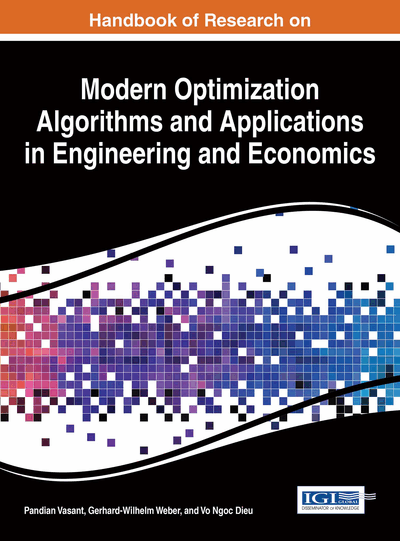# Modeling a Real Cable Production System as a Single Machine-Scheduling Problem: Mathematical Model and Metaheuristic Approach

Sadegh Niroomand (Firouzabad Institute of Higher Education, Iran) and Béla Vizvári (Eastern Mediterranean University, North Cyprus, Turkey)
DOI: 10.4018/978-1-4666-9644-0.ch012
Available
\$37.50
No Current Special Offers

## Abstract

In cases where the size and colour of cable are changed, the cable industry is classified as a multi-product, mass production system. The paper provides a mixed integer linear programming model based on continuous time representation for a case study on the scheduling problem of the cable industry to minimize the total cost including setup cost, operating cost, and inventory holding cost. As the solution methodology, three grouping policies are proposed while Xpress solver could not give any feasible solution for the model. Cables of the same size and the same colour, respectively, of the different types of cable are grouped together. A metaheuristic based on a simulated annealing algorithm is applied to minimize the total cost of proposed solutions. Finally the solution with the smallest total cost is selected as the production schedule of the study case.
Chapter Preview
Top

## 1. Introduction

In manufacturing organizations, goals like profitability, quality of products, on-time delivery, and so on play a critical role in keeping and increasing the market share of organizations. The scheduling of tasks in manufacturing helps to decrease production costs and to shorten the lead time of finished products. Classical scheduling problems are mostly time-based optimization problems, while the processing time and setup time of tasks are assumed to be constant. A well-known type of scheduling problem is known as the single machine scheduling problem, which concerns the arrangement of jobs on a single machine/system considering setup times and processing times of jobs. A good survey of scheduling problems with setup times is presented by Allahverdi et al. (2008).

Scheduling problems need efficient mathematical models in order to be solved effectively. Generally, mixed integer linear programming (MILP) models are used for such problems. Pinedo (2008) classifies these scheduling problems as complex optimization problems that belong to NP-hard problems. Floudas and Lin (2005) focused on the application of MILP in process scheduling while Barton and Lee (2004), Kallrath (1999), Lee and Grossman (2003) and Fahmy and ElMekkawy and Balakrishnan (2008) using mixed integer nonlinear programming (MINLP) to formulate scheduling problems. A mixed integer quadratic programming (MIQP) model was also applied by Dua and Morari (2004).

The mathematical models of scheduling problems are divided into two types, discrete and continuous formulations. In discrete formulation, the tasks must be started at the beginning or end of predetermined equal discrete time intervals. Floudas and Lin (2004) focused on the discrete formulation. These models need a large number of binary variables for representing the scheduling problem. They also represent an approximate time domain (Stefansson et al. (2011)). On the other hand, continuous formulation allows the tasks to be started at any time under the condition that there is no overlap with other tasks. Most of the researchers used continuous formulations to model scheduling problems, for example, Giannelos and Georgiadis (2002), Harjunkoski and Grossmann (2002), Janak and lin and Floudas (2005), Castro and Grossmann and Novais (2006), and others. Figure 1 shows the arrangement of tasks based on discrete and continuous models.

Different solution approaches, for example exact and non-exact, are used to solve the mathematical model of scheduling problems. A well-known exact approach is Branch and Bound’s method, which can be applied by heuristic algorithms or optimization software, for example Cplex, Xpress, Lingo, and so on. This method was applied by Rios-Mercado et al. (1999), Rabadi et al. (2004), Dunstall et al. (2005), Ranjbar (2013), Neto et al. (2013), and so on. Different metaheuristic algorithms, for example simulated annealing (SA), genetic algorithm, tabu search, ant colony, and so on, were proposed as non-exact methods in order to obtain a good feasible solution where the degree of difficulty of the model is high and exact methods are not able to solve the mathematical model in reasonable time. Studies of Gilland (2002), Kim et al. (2002), Mendes et al. (2002), Bilge et al. (2004), Low (2005), Dupuy et al. (2005), Ruiz et al. (2006), Hendizadeh et al. (2007), Valente (2007), Damodaran et al. (2009), Wong et al. (2012) and Yang and Shen (2012)Yousefi and Yusuff (2013) can be mentioned as authors who have applied metaheuristics to scheduling problems.

## Complete Chapter List

Search this Book:
Reset